Ex 12.1

Chapter 12 Class 10 Areas related to Circles
Serial order wise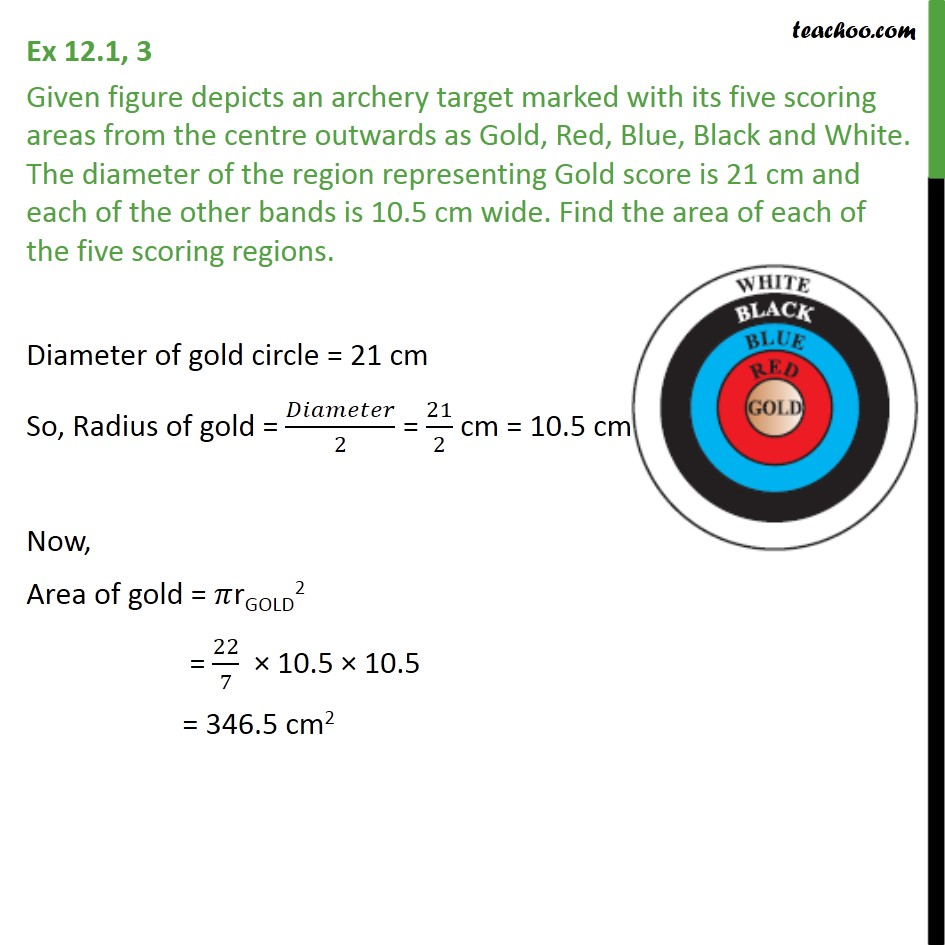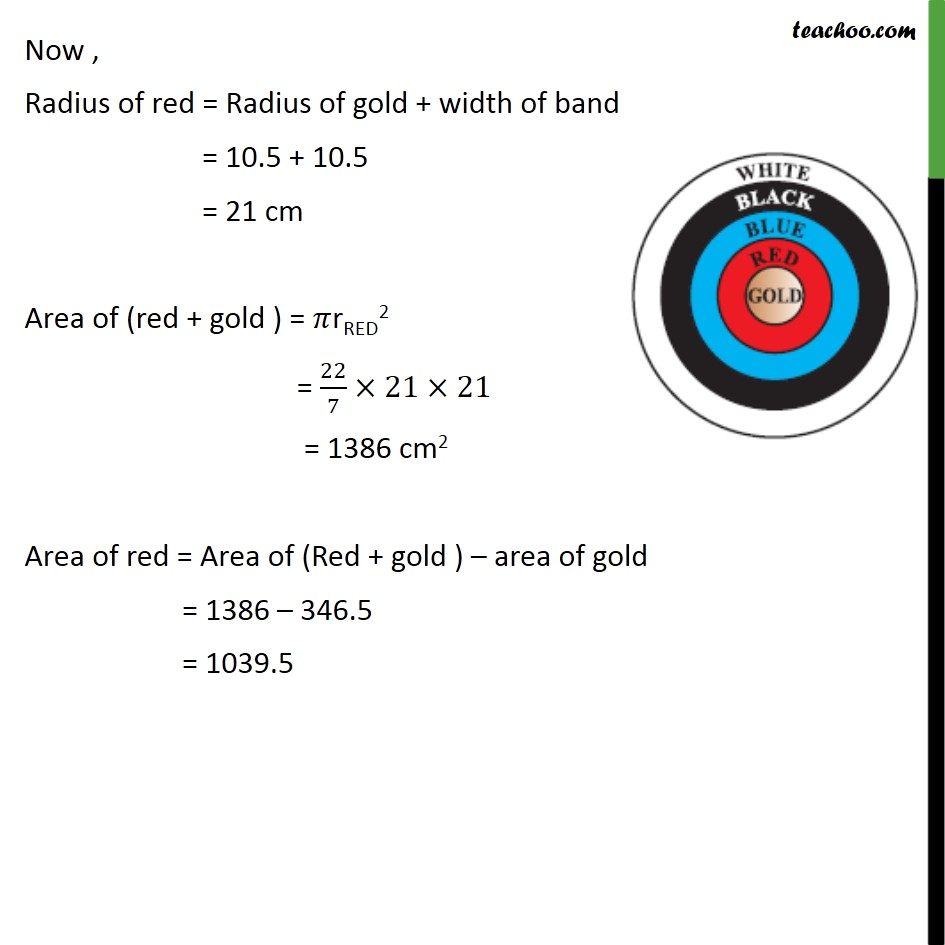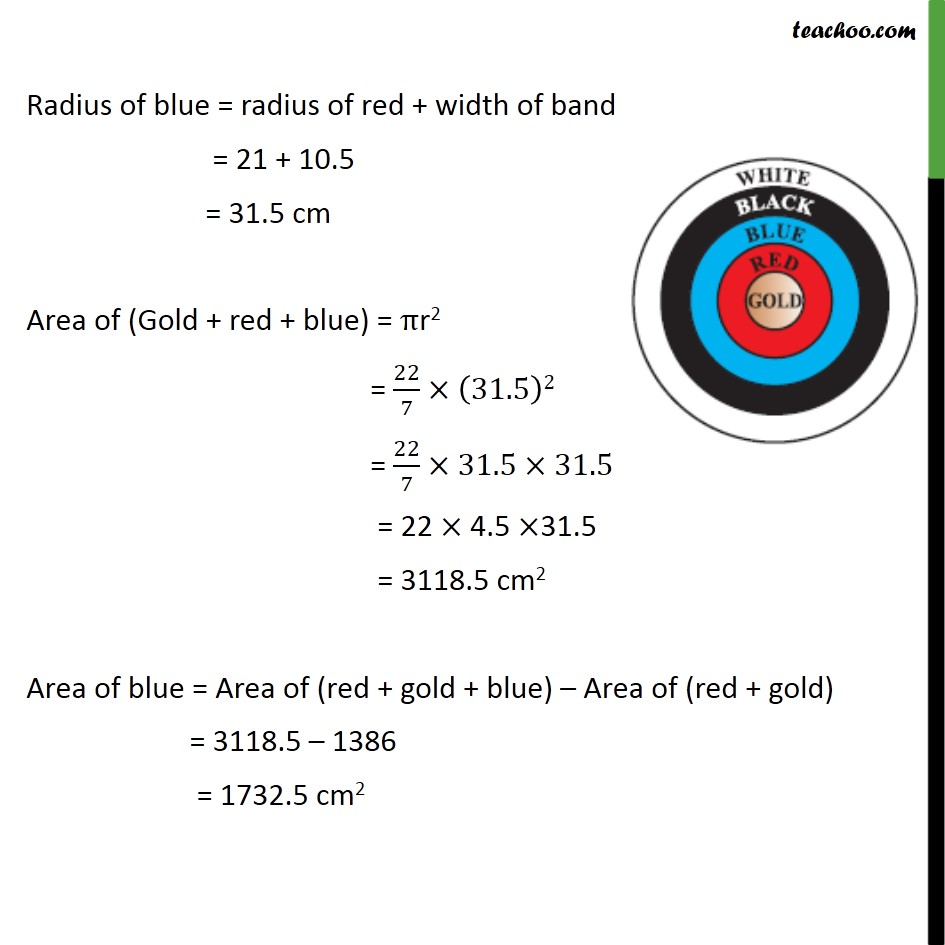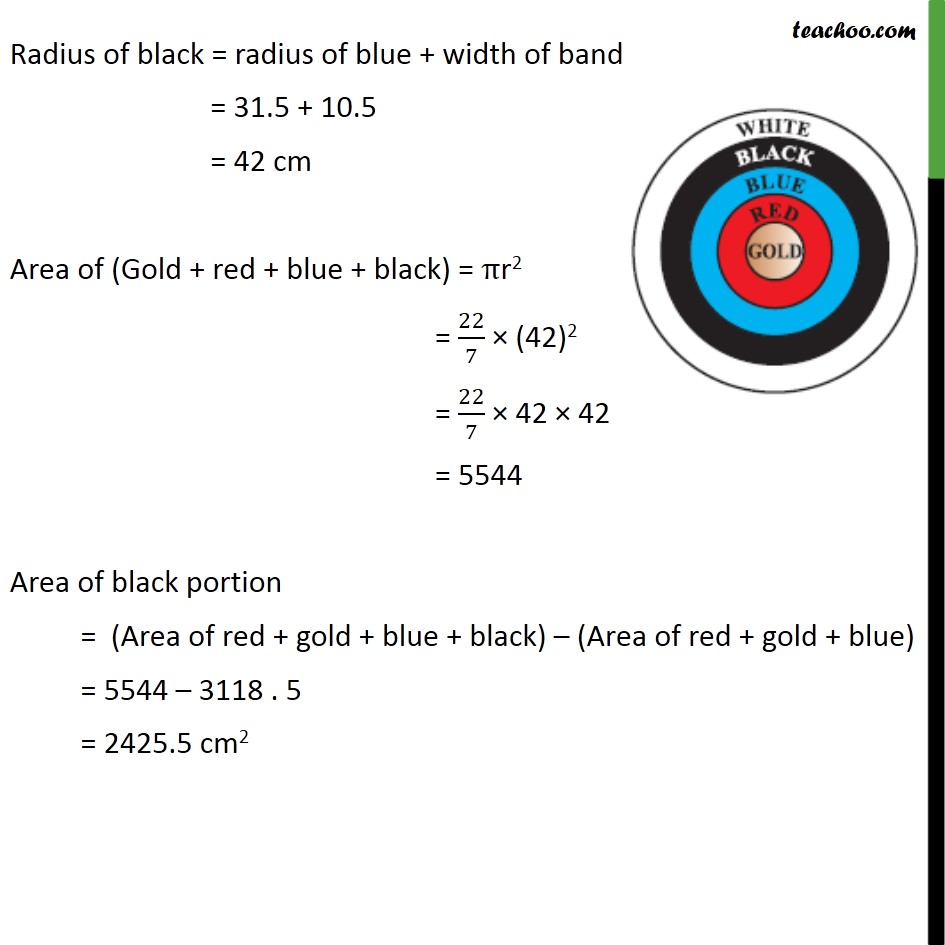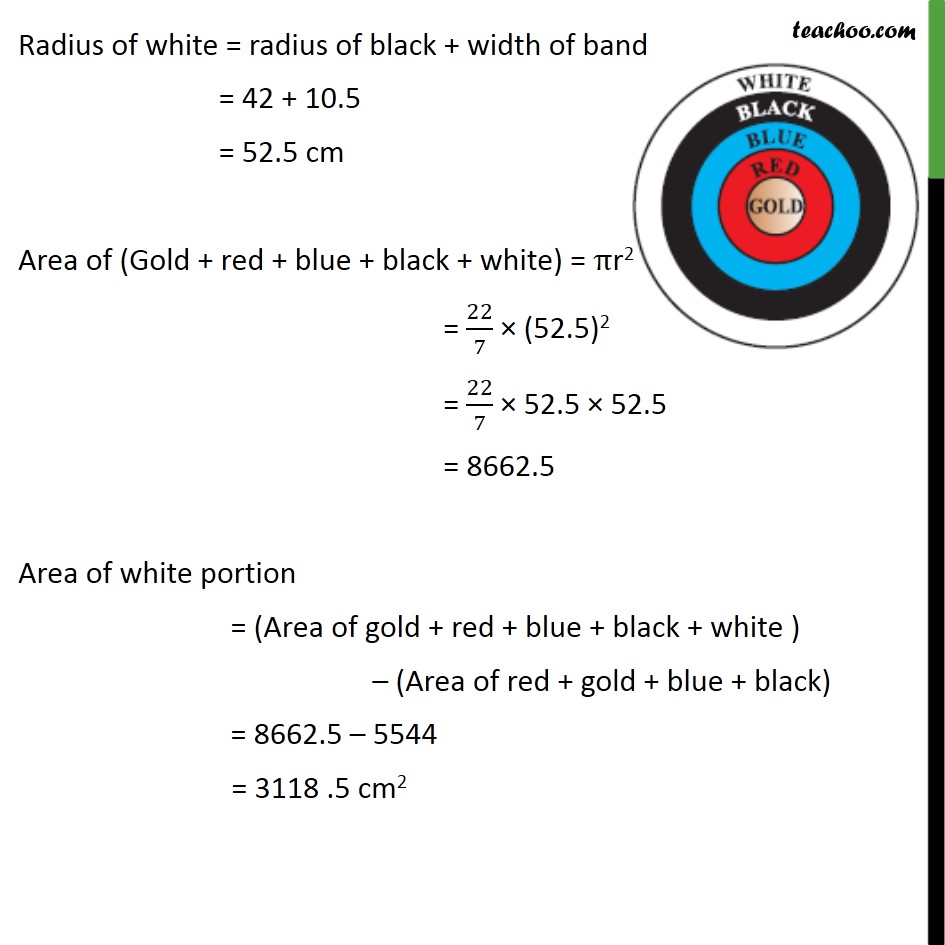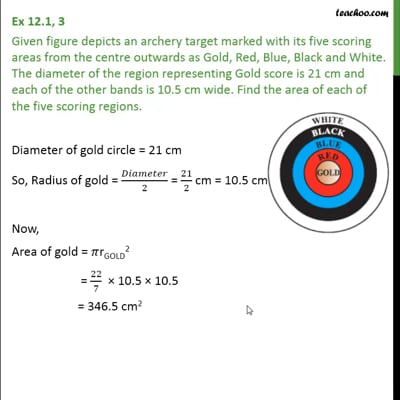This video is only available for Teachoo black users

Solve all your doubts with Teachoo Black (new monthly pack available now!)

### Transcript

Ex 12.1, 3 Given figure depicts an archery target marked with its five scoring areas from the centre outwards as Gold, Red, Blue, Black and White. The diameter of the region representing Gold score is 21 cm and each of the other bands is 10.5 cm wide. Find the area of each of the five scoring regions. Diameter of gold circle = 21 cm So, Radius of gold = /2 = 21/2 cm = 10.5 cm Now, Area of gold = rGOLD2 = 22/7 10.5 10.5 = 346.5 cm2 Now , Radius of red = Radius of gold + width of band = 10.5 + 10.5 = 21 cm Area of (red + gold ) = rRED2 = 22/7 21 21 = 1386 cm2 Area of red = Area of (Red + gold ) area of gold = 1386 346.5 = 1039.5 Radius of blue = radius of red + width of band = 21 + 10.5 = 31.5 cm Area of (Gold + red + blue) = r2 = 22/7 (31.5)2 = 22/7 31.5 31.5 = 22 4.5 31.5 = 3118.5 cm2 Area of blue = Area of (red + gold + blue) Area of (red + gold) = 3118.5 1386 = 1732.5 cm2 Radius of black = radius of blue + width of band = 31.5 + 10.5 = 42 cm Area of (Gold + red + blue + black) = r2 = 22/7 (42)2 = 22/7 42 42 = 5544 Area of black portion = (Area of red + gold + blue + black) (Area of red + gold + blue) = 5544 3118 . 5 = 2425.5 cm2 Radius of white = radius of black + width of band = 42 + 10.5 = 52.5 cm Area of (Gold + red + blue + black + white) = r2 = 22/7 (52.5)2 = 22/7 52.5 52.5 = 8662.5 Area of white portion = (Area of gold + red + blue + black + white ) (Area of red + gold + blue + black) = 8662.5 5544 = 3118 .5 cm2